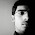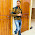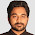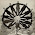# OpenGl code for collision detection between two Objects

This source code is for making two moving balls. They move in opposite side when they collide.

OpenGL program:-

```#ifdef __APPLE__
#include<GLUT/glut.h>
#include<openGL/openGL.h>

#else
#include<GL/glut.h>
#endif

float x1=-2.0,x2=2.0;
static int flag=1;```

```void initRendering()
{
glEnable(GL_DEPTH_TEST);
}

void reshape(int w,int h)
{
glViewport(0,0,w,h);

glMatrixMode(GL_PROJECTION);

gluPerspective(45,w/h,1,200);

}

void keyPressed(int key,int x,int y)
{
if(key==GLUT_KEY_LEFT)
{}
}

void drawBall1()
{
glColor3f(1.0,0.0,0.0);

glPushMatrix();

glTranslatef(x1,0.0,-5.0);

glutSolidSphere(0.4,20,20);

glPopMatrix();
}

void drawBall2()
{
glColor3f(0.0,0.0,1.0);

glPushMatrix();

glTranslatef(x2,0.0,-5.0);

glutSolidSphere(0.4,20,20);

glPopMatrix();

}

void update()
{
if(flag)
{
x1+=0.005;
x2-=0.005;
if(x1>-0.35)
flag=0;
}
if(!flag)
{
x1-=0.005;
x2+=0.005;
if(x1<-2.0)
flag=1;
}
}```

```void display()
{
glClear(GL_COLOR_BUFFER_BIT|GL_DEPTH_BUFFER_BIT);

glMatrixMode(GL_MODELVIEW);

drawBall1();

drawBall2();

update();

glutSwapBuffers();
}

int main(int argc,char **argv)
{
glutInit(&argc,argv);

glutInitDisplayMode(GLUT_DOUBLE|GLUT_RGB|GLUT_DEPTH);

glutInitWindowSize(400,400);

glutCreateWindow("Collision Window");

initRendering();

glutDisplayFunc(display);

glutIdleFunc(display);

glutReshapeFunc(reshape);

glutSpecialFunc(keyPressed);

glutMainLoop();

return(0);
}
```

//Output of the above Program:-

Related Programs:-

Simple Triangles

Simple Triangle and Bouncing Ball

Simple Cloud

Moving Circle

Moving Car

1.great work thanks for this post

1.Thanks bro.. :)

2.how to run ??

2.Thank You so much brother ..Great post ..Helped me a lot for my project. Good Luck

3.Hi, I edited this code based on what I've learned about sphere-sphere collision detection. The key to sphere-sphere collision detection is to check if sum of their radius is greater then distance between their middle points, and not just their position as it is done in the original code. For less operations to take place, we use square values. I added ball-wall collision, where wall is not a polygon, but simple plane(not visible). Note that each ball should be paired with each ball and each wall (at the moment it is not so). Adding more balls is now simpler because of new Ball structure. But, adding more and more balls and walls would require some kind of array and space structure to handle them all. Hope this helps someone: http://pastebin.com/H572Fgpf